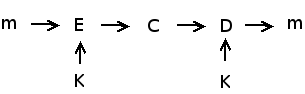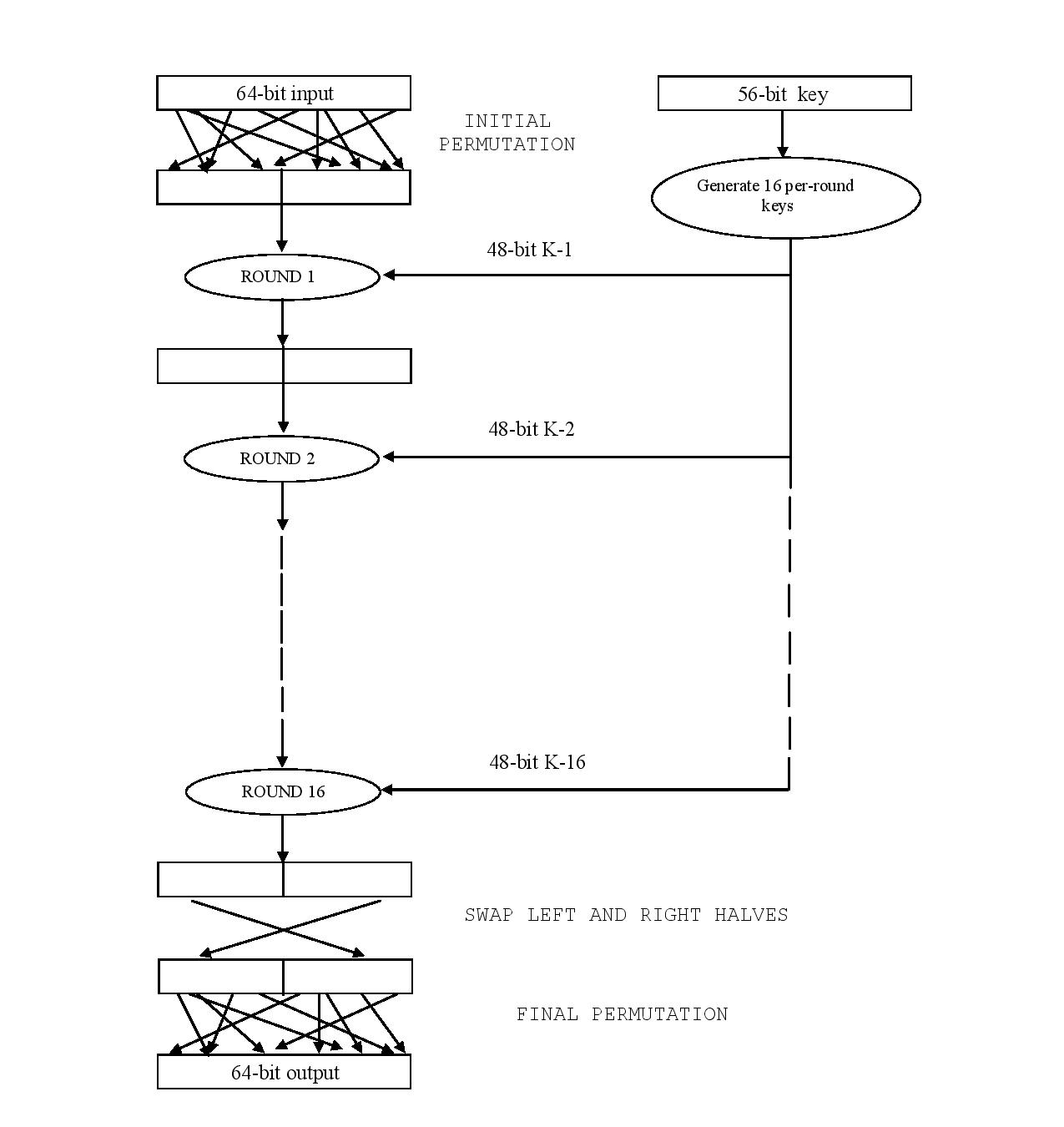life is too short for a diary

# Data Encryption Standard (DES)

Data Encryption Standard, a symmetric key algorithm, implemented in C++. All the parties use the same key for encryption and decryption. Look at the diagram below.Here, m is message which is passed through an encrypted function E along with key K. The resultant is a unintelligent gibberish cipher. The cipher is decoded again using the same key to produce plain message.

Though DES is not used nowdays in many application, it was the predominant algorithm developed by IBM (and later modified by NSA) for encrypting electronic data. However Triple DES is considered secure and is still used in many application.

DES is a Block Cipher. DES works on 64 bits blocks. Hence the plaintext is divided into chunks of 64 bits. Padding is used if the data could not be divided into equal blocks.

The key used is of 56 bits length which is extracted from the 64 bit original key (by ignoring every eight bit).

Description

After initial permutation, block of 64 bits of plaintext is broken into right and left half of 32 bits each. It is followed by 16 rounds of identical operations called Function f. In this the data are algorithmically combined with the key.

In each round, the key bits are shifted and then 48 bits are selected from 56 bits of key. The right half of data is expanded to 48 bits via an expansion permutation, combined with 48 bits of a shifted and permuted key via an XOR, sent through 8 s-boxes producing 32 new bits and permuted again. The four operation make up Function f. The output of Function f is then combined with the left half via another XOR operation. The result of these operation becomes the new left half. Mathematically Function f could be written as

```<pre> Li = Ri - 1 Ri = Li - 1 ⊕ f(Ri - 1, Ki) </pre>```

After 16th round, right and left halves are joined and a final permutation finishes off the algorithm. To better understand, look at the diagram below. 1Conclusion

I implemented this project in C++. This was the first encryption standard which I studied and implemented.

1. Network Security: Private Communication in a Public World